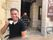cancel
Showing results for
Did you mean:## Dynamically Detect Column Types with Power M

The below Power M function will take a table as a parameter and return a table where the data types have been set.

Please mind, it currently only works for the datatypes; datetime, date, number and text. This should not be an issue for those who are skilled in M, since you can easily extend the function.

Pls also mind the final row in the code, here you see that fn is called upon whereby a table is passed, but also the number of rows and the allowed error margin.

Credits to Cris Webb for explaining how Expression. Evaluate works. Credits to Imke for explaining how the transformcolumn types work.

``````(tbl as table) as table  =>
let
fn = (tbl as table, col as text, numberofrecords as number, marginforerror as number) as type =>
let
LijstmetValues = List.FirstN( Table.Column(tbl, col),numberofrecords),
Env = Record.Combine({[L=LijstmetValues],[DTF = #shared[DateTime.From]], [DF = #shared[Date.From]], [NF = #shared[Number.From]], [TF = #shared[Text.From]], [LT = #shared[List.Transform]], [LS = #shared[List.Select]], [LC = #shared[List.Count]]   }),
NumberOfErrors = List.Transform({"DTF", "DF", "NF", "TF"}, each Expression.Evaluate("
LC(LS(
LT(L, each try  " & _ & "(_) otherwise ""Error""), each _ = ""Error""))", Env)),
CheckWithinMargin = List.Transform(NumberOfErrors, each _ <= numberofrecords * marginforerror),
typenr = List.PositionOf(CheckWithinMargin, true),

FirstTypeWithinMargin =  {"datetime", "date", "number", "text"}{typenr},
CheckType = if List.Distinct(LijstmetValues){0} = null or FirstTypeWithinMargin = -1 then 4  else FirstTypeWithinMargin,
result = Record.Field([number = type number,date = type date,datetime = type datetime,text = type text, any = type any],CheckType)
in result,
Columnsto2Type = Table.TransformColumnTypes(tbl, List.Transform(Table.ColumnNames(tbl), each {_,  fn(tbl,_, 7, 0.1)}))
in
Columnsto2Type``````

The animation below hopefully clarifies how you could potentially use this code. Notice that I removed the steps that automatically detected the datatypes (when creating the table initially), so the function can take on this task when we are manipulating multiple tables. This should demonstrate some more advanced use cases where we need to detect data types.

The example file is attached.

Kind regards, Steve.